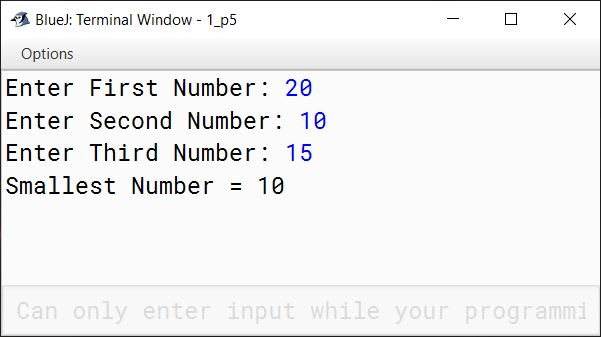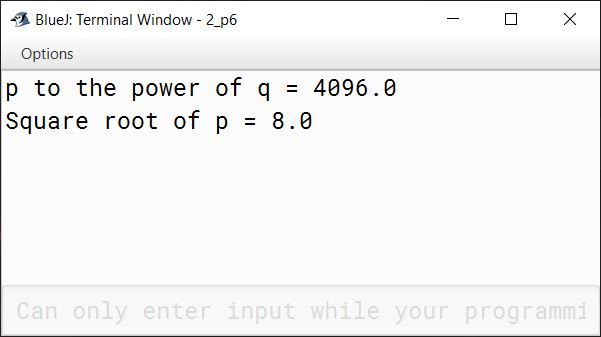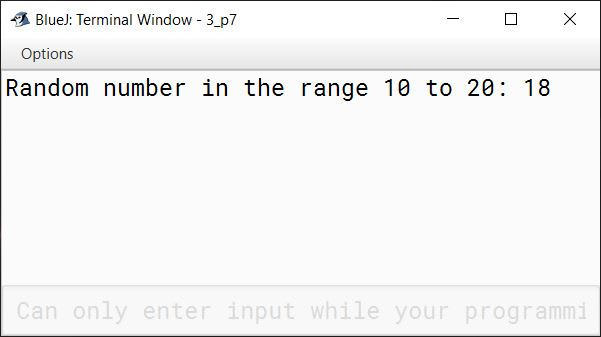# Mathematical Library Methods

## Multiple Choice Questions

#### Question 1

Which Java package includes the Math class?

1. java.io
2. java.lang ✓
3. java.util
4. java.sys

#### Question 2

Give the output of Math.sqrt(x); when x = 9.0

1. 3
2. 3.0 ✓
3. 3.00
4. All of these

#### Question 3

Give the output of Math.ceil(46.6).

1. 46.6
2. 46.5
3. 47.0 ✓
4. 46.0

#### Question 4

Give the output of Math.abs(x); when x = -9.99

1. -9.99
2. 9.99 ✓
3. 0.99
4. None of these

#### Question 5

Which of the following is a method to find the square root of a number?

1. FindSquareroot(x)
2. Sqrt(x)
3. Math.Square(x)
4. Math.sqrt(x) ✓

#### Question 6

What will be the output of Math.pow(2, 0)?

1. 0.0
2. 1.0 ✓
3. 2.0
4. -1.0

#### Question 7

Math.random() returns a double value r such that

1. 0.0 <= r < 1.0 ✓
2. 0.0 <= r <= 1.0
3. 0.0 < r <= 1.0
4. 0.0 < r < 1.0

#### Question 8

Give the output of Math.floor(46.6)?

1. 46.5
2. 47.0
3. -46.0
4. 46.0 ✓

## Assignment Questions

#### Question 1

Distinguish between Math.ceil() and Math.floor() methods.

Math.ceil( )Math.floor( )
Returns the smallest double value that is greater than or equal to the argument and is equal to a mathematical integerReturns the largest double value that is less than or equal to the argument and is equal to a mathematical integer.
double a = Math.ceil(65.5);
In this example, a will be assigned the value of 66.0 as it is the smallest integer greater than 65.5.
double b = Math.floor(65.5);
In this example, b will be assigned the value of 65.0 as it is the largest integer smaller than 65.5.

#### Question 2

Explain the following Math functions in Java:

i. Math.sqrt()

Returns the square root of its argument as a double value. For example, Math.sqrt(25) will return 5.0.

ii. Math.cbrt()

Returns the cube root of its argument as a double value. For example, Math.cbrt(27) will return 3.0.

iii. Math.random()

Returns a positive double value, greater than or equal to 0.0 and less than 1.0.

iv. Math.round()

Rounds off its argument to the nearest mathematical integer and returns its value as an int or long type. If argument is float, return type is int, if argument is double, return type is long. At mid-point, it returns the higher integer. For example, Math.round(2.5) will return 3.

#### Question 3

Write the following as Java expressions:

i. $\lvert x^3 - y^2 -2xy\rvert$

Math.abs(Math.pow(x, 3) - Math.pow(y, 2) - 2*x*y)

ii. $\dfrac{\pi}{6}(z^4 - 2\pi)$

Math.PI / 6*(Math.pow(z, 4) - 2*Math.PI)

iii. $\sqrt{z^2 - \pi}$

Math.cbrt(z*z - Math.PI)

iv. $\sqrt{x^3 - y^3}$

Math.pow(x*x*x - y*y*y, 1/4)

v. $\cfrac{amount*rate}{1 - \cfrac{1}{(1 + rate)^n}}$

(amount*rate) / (1 - (1 / Math.pow(1+rate, n)))

#### Question 4

Write valid statements for the following in Java:

i. Print the rounded off value of 234.49

System.out.println(Math.round(234.49));

ii. Print the absolute value of -9.99

System.out.println(Math.abs(-9.99));

iii. Print the largest of -45 and -50

System.out.println(Math.max(-45, -50));

iv. Print the smallest of -56 and -57.4

System.out.println(Math.min(-56, -57.4));

v. Print a random integer between 50 and 70

int range = 70 - 50 + 1;
int num = (int)(range * Math.random() + 50);
System.out.println(num);

vi. Print 10.5 raised to the power 3.8

System.out.println(Math.pow(10.5, 3.8));

#### Question 5

Write a program in Java to find the minimum of three numbers using Math.min() method.

import java.util.Scanner;

public class KboatSmallestNumber
{
public static void main(String args[]) {

Scanner in = new Scanner(System.in);

System.out.print("Enter First Number: ");
int a = in.nextInt();
System.out.print("Enter Second Number: ");
int b = in.nextInt();
System.out.print("Enter Third Number: ");
int c = in.nextInt();

int s = Math.min(a, b);
s = Math.min(s, c);

System.out.println("Smallest Number = " + s);
}
}
##### Output#### Question 6

Write a program to print:

i. p to the power q
ii. the square root of p

The values of p and q are 64 and 2 respectively.

public class KboatNumber
{
public static void main(String args[]) {
int p = 64;
int q = 2;

double r1 = Math.pow(p, q);
System.out.println("p to the power of q = " + r1);

double r2 = Math.sqrt(p);
System.out.println("Square root of p = " + r2);
}
}
##### Output#### Question 7

Write a program to generate random integers in the range 10 to 20.

public class KboatRandom
{
public static void main(String args[]) {
int min = 10, max = 20;
int range = max - min + 1;
int num = (int)(range * Math.random() + min);
System.out.println("Random number in the range 10 to 20: " + num);
}
}
##### Output#### Question 8

Write the equivalent Java statements for the following, only using the mathematical functions:

i. Print the positive value of -101.

System.out.println(Math.abs(-101));

ii. Store the value -125 in a variable and print its cube root.

int a = -125;
System.out.println(Math.cbrt(a));
double a = 89.99;
System.out.println(Math.round(a));